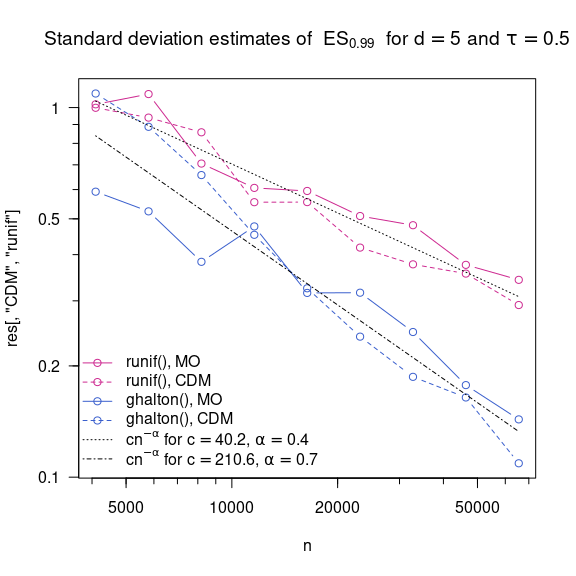Quasi-Random Numbers for Copula Models

2020-12-11

In this vignette, we present some findings around quasi-random numbers for copula models; see also Cambou et al. (2016, “Quasi-random numbers for copula models”). Note that not all plots are displayed (to keep the tarball small).

library(lattice)
library(copula)
library(VGAM)
library(gridExtra)
library(qrng)
library(randtoolbox)

1 Quasi-random numbers for copula models via conditional distribution method

Independence copula

n <- 1000 # sample size
set.seed(271) # set the seed (for reproducibility)
U <- matrix(runif(n*2), ncol = 2) # pseudo-random numbers
U. <- halton(n, dim = 2) # quasi-random numbers
par(pty = "s", mfrow = 1:2)
plot(U,  xlab = expression(italic(U)*"'"), ylab = expression(italic(U)*"'"))
plot(U., xlab = expression(italic(U)*"'"), ylab = expression(italic(U)*"'"))

Let’s check if the more equally spaced points (less gaps, less clusters) are preserved in the copula world when determined with one-to-one transformations (such as the conditional distribution method (CDM); this can be obtained via cCopula(, inverse=TRUE)).

Clayton copula

Consider a Clayton copula.

family <- "Clayton"
tau <- 0.5
th <- iTau(getAcop(family), tau)
cop <- onacopulaL(family, nacList = list(th, 1:2))
U.C  <- cCopula(U,  copula = cop, inverse = TRUE) # via PRNG
U.C. <- cCopula(U., copula = cop, inverse = TRUE) # via QRNG
par(pty = "s", mfrow = 1:2)
plot(U.C,  xlab = expression(italic(U)), ylab = expression(italic(U)), cex = 0.4)
plot(U.C., xlab = expression(italic(U)), ylab = expression(italic(U)), cex = 0.4)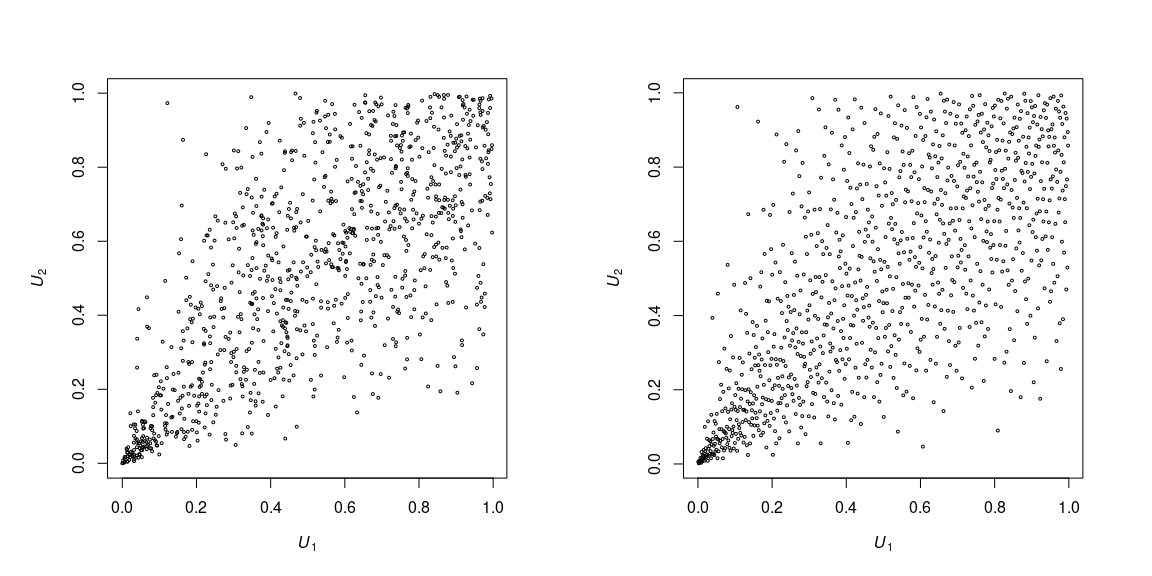$$t$$ copula with three degrees of freedom

Consider a $$t$$ copula with three degrees of freedom.

family <- "t"
nu <- 3 # degrees of freedom
tau <- 0.5 # Kendall's tau (determines the copula parameter rho)
th <- iTau(ellipCopula(family, df = nu), tau)
cop <- ellipCopula(family, param = th, df = nu)
U.t  <- cCopula(U,  copula = cop, inverse = TRUE) # via PRNG
U.t. <- cCopula(U., copula = cop, inverse = TRUE) # via QRNG
par(pty = "s", mfrow = 1:2)
plot(U.t,  xlab = expression(italic(U)), ylab = expression(italic(U)), cex = 0.4)
plot(U.t., xlab = expression(italic(U)), ylab = expression(italic(U)), cex = 0.4)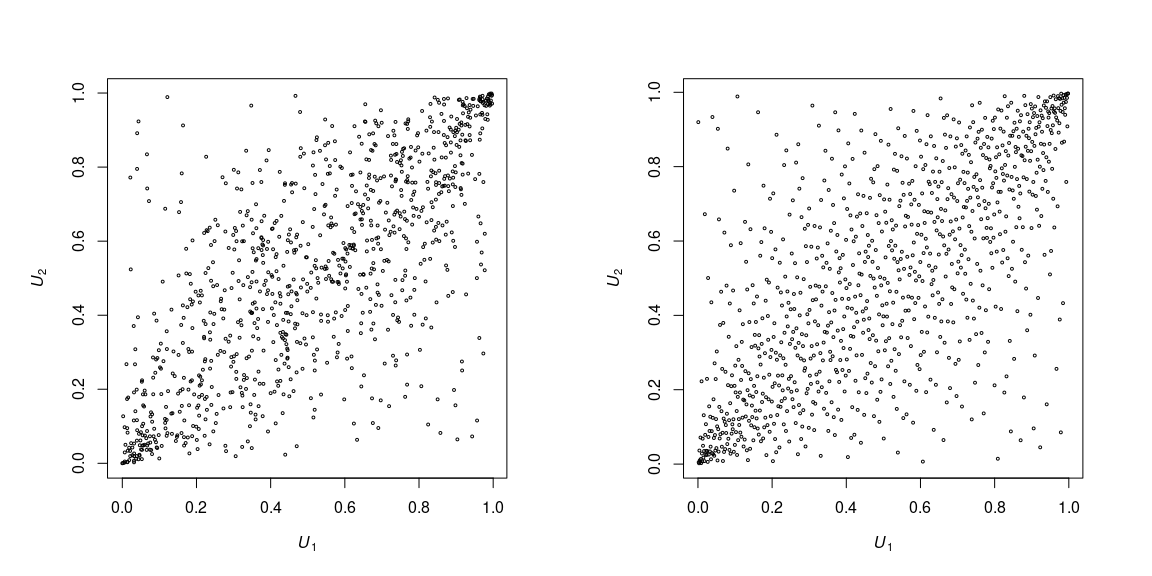Marshall–Olkin copula

Now something more fancy, a Marshall–Olkin copula.

alpha <- c(0.25, 0.75)
tau <- (alpha*alpha) / (alpha+alpha-alpha*alpha)
##' @title Inverse of the bivariate conditional Marshall--Olkin copula
##' @param u (n,2) matrix of U[0,1] random numbers to be transformed to
##'        (u[,1], C^-(u[,2]|u[,1]))
##' @param alpha bivariate parameter vector
##' @return (u[,1], C^-(u[,2]|u[,1])) for C being a MO copula
##' @author Marius Hofert
inv_cond_cop_MO <- function(u, alpha)
{
stopifnot(is.matrix(u), 0 <= alpha, alpha <= 1)
up <- u[,1]^(alpha*(1/alpha-1))
low <- (1-alpha)*up
i1 <- u[,2] <= low
i3 <- u[,2] >  up
u2 <- u[,1]^(alpha/alpha)
u2[i1] <- u[i1,1]^alpha * u[i1,2] / (1-alpha)
u2[i3] <- u[i3,2]^(1/(1-alpha))
cbind(u[,1], u2)
}
U.MO  <- inv_cond_cop_MO(U,  alpha = alpha)
U.MO. <- inv_cond_cop_MO(U., alpha = alpha)
par(pty = "s", mfrow = 1:2)
plot(U.MO,  xlab = expression(italic(U)), ylab = expression(italic(U)), cex = 0.4)
plot(U.MO., xlab = expression(italic(U)), ylab = expression(italic(U)), cex = 0.4)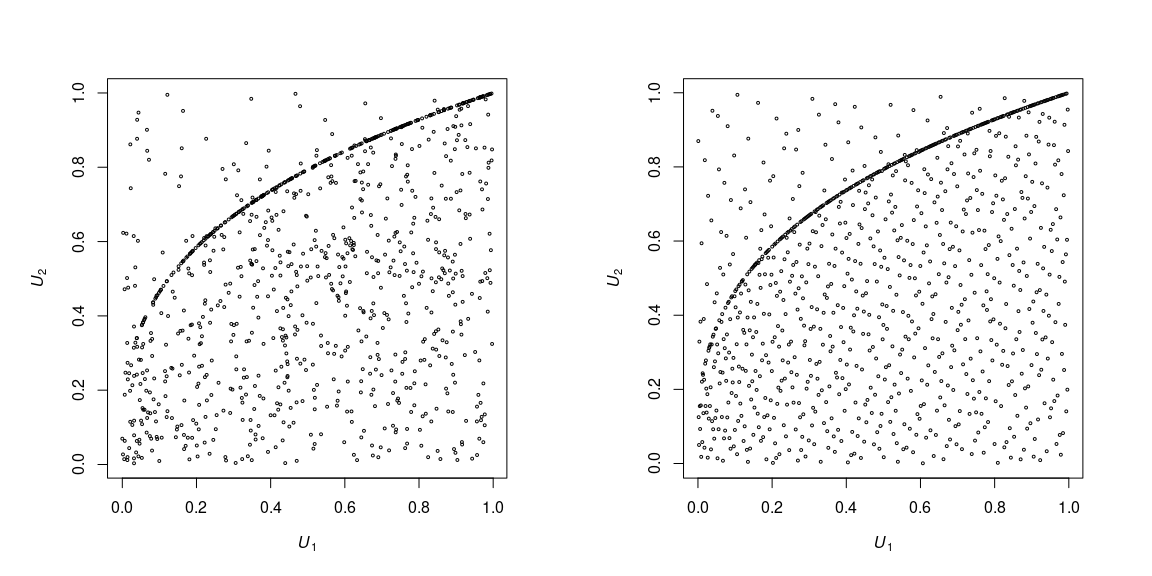3d $$t$$ copula with three degrees of freedom

Let’s consider a three-dimensional $$t$$ copula with three degrees of freedom.

family <- "t"
nu <- 3 # degrees of freedom
tau <- 0.5 # Kendall's tau (determines the copula parameter rho)
th <- iTau(ellipCopula(family, df = nu), tau)
cop <- ellipCopula(family, param = th, dim = 3, df = nu)

First the pseudo-random version.

U.3d <- matrix(runif(n*3), ncol = 3)
U.t.3d <- cCopula(U.3d, copula = cop, inverse = TRUE)
par(pty = "s")
pairs(U.t.3d, gap = 0,
labels = as.expression(sapply(1:3, function(j) bquote(italic(U[.(j)])))))

Now the quasi-random version.

U.3d. <- halton(n, dim = 3)
U.t.3d. <- cCopula(U.3d., copula = cop, inverse = TRUE)
par(pty = "s")
pairs(U.t.3d., gap = 0,
labels = as.expression(sapply(1:3, function(j) bquote(italic(U[.(j)])))))

Note that projections (here: to pairs) can appear not to be quasi-random’ (or appear not to possess a lower discrepancy), but see Section 2.2 below! Visualization in more than two dimensions seems difficult; we have just seen bivariate projections and ‘quasi-randomness’ is also not easily visible from a 3d cloud plot.

p1 <- cloud(U.t.3d[,3]~U.t.3d[,1]+U.t.3d[,2], scales = list(col = 1, arrows = FALSE),
col = 1, xlab = expression(italic(U)), ylab = expression(italic(U)),
zlab = expression(italic(U)),
par.settings = list(background = list(col = "#ffffff00"),
axis.line = list(col = "transparent"),
clip = list(panel = "off")))
p2 <- cloud(U.t.3d.[,3]~U.t.3d.[,1]+U.t.3d.[,2], scales = list(col = 1, arrows = FALSE),
col = 1, xlab = expression(italic(U)), ylab = expression(italic(U)),
zlab = expression(italic(U)),
par.settings = list(background = list(col = "#ffffff00"),
axis.line = list(col = "transparent"),
clip = list(panel = "off")))
grid.arrange(p1, p2, ncol = 2)

3d R-Vine copula

As another example, consider a three-dimensional R-vine copula. Note that this is not run here to avoid a cyclic dependency (since VineCopula imports copula).

if(FALSE) {
library(VineCopula)
M <- matrix(c(3, 1, 2,
0, 2, 1,
0, 0, 1), ncol = 3) # R-vine tree structure matrix
family <- matrix(c(0, 3, 3, # C, C
0, 0, 3, #    C
0, 0, 0), ncol = 3) # R-vine pair-copula family matrix (0 = Pi)
param <- matrix(c(0, 1, 1,
0, 0, 1,
0, 0, 0), ncol = 3) # R-vine pair-copula parameter matrix
param. <- matrix(0, nrow = 3, ncol = 3) # 2nd R-vine pair-copula parameter matrix
RVM <- RVineMatrix(Matrix = M, family = family, par = param, par2 = param.) # RVineMatrix object
## First the pseudo-random version
U <- RVineSim(n, RVM) # PRNG
par(pty = "s")
pairs(U, labels = as.expression( sapply(1:3, function(j) bquote(italic(U[.(j)]))) ),
gap = 0, cex = 0.3)
## Now the quasi-random version
U. <- RVineSim(n, RVM, U = halton(n, d = 3)) # QRNG
par(pty = "s")
pairs(U., labels = as.expression( sapply(1:3, function(j) bquote(italic(U[.(j)]))) ),
gap = 0, cex = 0.3)
## Similarly to the 3d *t* copula case (because of the projections to pairs),
## not all pairs appear to be 'quasi-random'.
}

2 Quasi-random numbers for copula models via stochastic representations

For many copula families, it is rarely efficient to sample them via the CDM (or other one-to-one transformations), one typically uses stochastic representations based on simple, easy-to-sample distributions as building blocks. Although, again, not directly visible, quasi-random numbers can also improve the low-discrepancy of the resulting random numbers and thus be used for variance reduction in the context of dependence.

2.1 Colorized scatter plot

To explore this, we sample from a Clayton copula via the CDM (so via a one-to-one transformation) and via the Marshall–Olkin algorithm (so via a stochastic representation in terms of the Gamma frailty distribution and two standard exponentials) based on a three-dimensional Halton sequence.

n <- 1000
family <- "Clayton"
tau <- 0.5
th <- iTau(getAcop(family), tau)
cop <- onacopulaL(family, nacList = list(th, 1:2))
## Generate dependent samples
U <- halton(n, 3)
U_CDM <- cCopula(U[,1:2], copula = cop, inverse = TRUE) # via CDM
U_MO <- copClayton@psi(-log(U[,2:3]) / qgamma(U[,1], 1/th), theta = th) # via Marshall-Olkin (MO)

## Colorization of U[,1:2]
col <- rep("black", n)
col[U[,1] <= 0.5 & U[,2] <= 0.5] <- "maroon3"
col[U[,1] >= 0.5 & U[,2] >= 0.5] <- "royalblue3"

## Colorization of U[,1:3] (= U)
col. <- rep("black", n)
col.[apply(U <= 0.5, 1, all)] <- "maroon3"
col.[apply(U >= 0.5, 1, all)] <- "royalblue3"

Colorized scatter plot (quasi-random numbers and CDM)

par(pty = "s", mfrow = 1:2)
plot(U[,1:2], xlab = expression(italic(U)*"'"), ylab = expression(italic(U)*"'"),
col = col, cex = 0.4)
plot(U_CDM,   xlab = expression(italic(U)), ylab = expression(italic(U)),
col = col, cex = 0.4)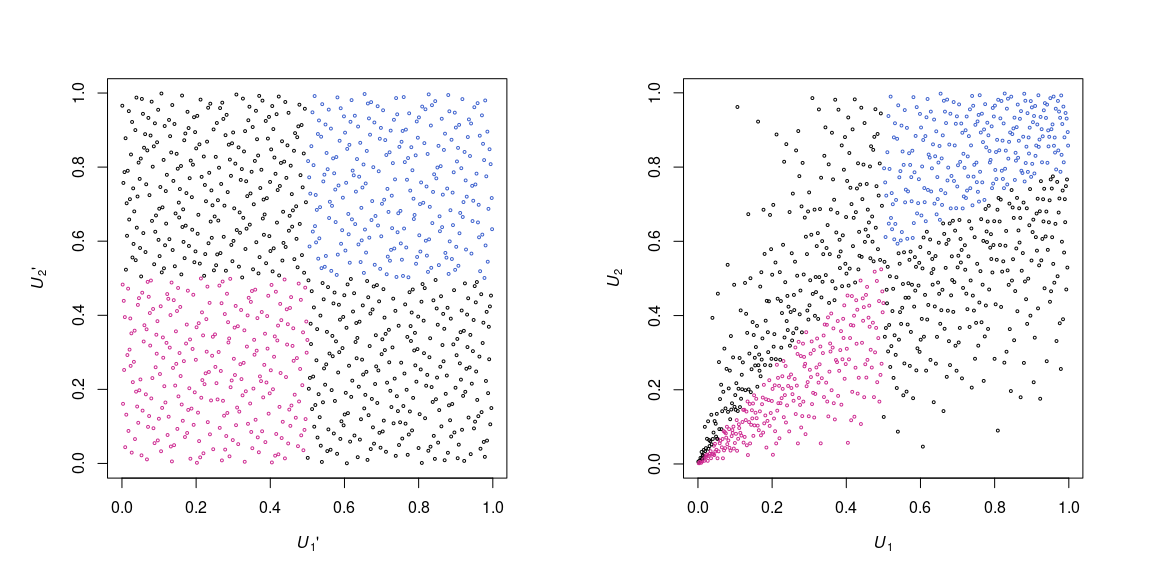Colorized scatter plots (quasi-random numbers and MO)

par(pty = "s", mfrow = 1:2)
plot(U[,2:3], xlab = expression(italic(U)*"'"), ylab = expression(italic(U)*"'"),
col = col., cex = 0.4)
plot(U_MO, xlab = expression(italic(U)), ylab = expression(italic(U)),
col = col., cex = 0.4)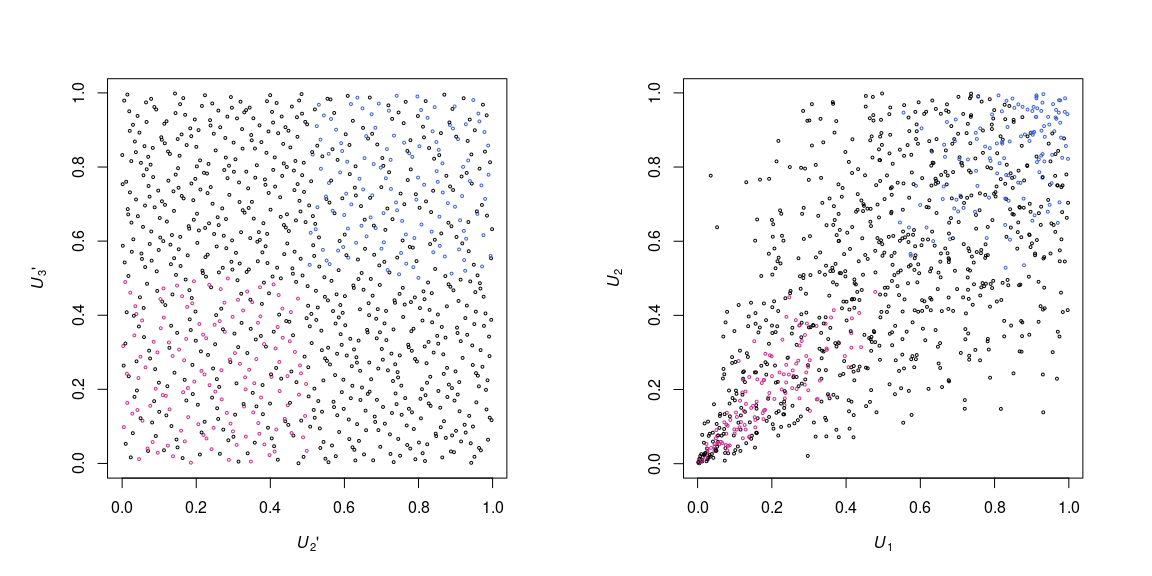2.2 A variance-reduction example

In this example, we would like to investigate the standard deviation when estimating expected shortfall at $$\alpha=99\%$$ confidence level via Monte Carlo simulation based on a Clayton copula with Pareto margins. To this end we consider pseudo-random numbers and quasi-random numbers, as well as two different sampling methods for the Clayton copula (the conditional distribution method and the Marshall–Olkin method (based on a well-known stochastic representation)), hence four different sampling methods.

Here is our setup.

n <- round(2^seq(12, 16, by = 0.5)) # sample sizes
B <- 25 # number of replications
d <- 5 # dimension
tau <- 0.5 # Kendall's tau
theta <- iTau(getAcop("Clayton"), tau) # copula parameter
qPar <- function(p, theta = 3) (1-p)^(-1/theta)-1 # marginal Pareto quantile function
rng.methods <- c("runif", "ghalton") # random number generation methods
cop.methods <- c("CDM", "MO") # copula sampling methods (conditional distribution method and Marshall--Olkin)
alpha <- 0.99 # confidence level

Next, let’s implement a function which can sample the Clayton copula with one of the four approaches.

##' @title Pseudo-/quasi-random number generation for (survival) Clayton copulas
##' @param n Sample size
##' @param d Dimension
##' @param B Number of replications
##' @param theta Clayton parameter
##' @param survival Logicial indicating whether a sample from the survival copula
##'        should be returned
##' @param rng.method Pseudo-/quasi-random number generator
##' @param cop.method Method to construct the pseudo-/quasi-random copula sample
##' @return (n, d, B)-array of pseudo-/quasi-random copula sample
##' @author Marius Hofert
rng_Clayton <- function(n, d, B, theta, survival = FALSE,
rng.method = c("runif", "ghalton"),
cop.method = c("CDM", "MO"))
{
## Sanity checks
stopifnot(n >= 1, d >= 2, B >= 1, is.logical(survival))
rng.method <- match.arg(rng.method)
cop.method <- match.arg(cop.method)

## Draw U(0,1) random numbers
k <- if(cop.method == "CDM") d else d+1
U. <- switch(rng.method,
"runif" = {
array(runif(n*k*B), dim = c(n,k,B)) # (n, k, B)-array
},
"ghalton" = {
replicate(B, expr = ghalton(n, d = k)) # (n, k, B)-array
},
stop("Wrong 'rng.method'"))

## Convert to pseudo-/quasi-random copula samples
U <- switch(cop.method, # B-list of (n, d)-matrices
"CDM" = {
cop <- onacopulaL("Clayton", nacList = list(theta, 1:d)) # d = k here
lst <- apply(U., 3, FUN = function(x) list(cCopula(x, copula = cop, inverse = TRUE)))
lapply(lst, [[`, 1)
},
"MO" = {
lapply(1:B, function(b) {
copClayton@psi(-log(U.[,2:k,b]) / qgamma(U.[,1,b], 1/theta), theta = theta)
})
},
stop("Wrong 'cop.method'"))

## Return
if(survival) 1-U else U # B-list of (n, d)-matrices
}

We also need an estimator of expected shortfall; we use the empirical estimator here.

ES <- function(x, alpha) mean(x[x > quantile(x, probs = alpha, type = 1)])

For each of the four methods, each of the chosen sample sizes and the number $$B$$ of (bootstrap) replications considered here, generated the samples, aggregate them and compute expected shortfall at the 99% confidence level. To reduce increase comparability, note that samples with smaller sample size are subsets of samples with larger sample size.

set.seed(271)
res.ES <- array(, dim = c(length(n), length(cop.methods), length(rng.methods), B),
dimnames = list(n = n, cop.meth = cop.methods, rng.meth = rng.methods, B = 1:B))
for(cmeth in cop.methods) {
for(rmeth in rng.methods) {
## Generate Clayton dependent random numbers with Par(3) margins
U <- rng_Clayton(max(n), d = d, B = B, theta = theta,
rng.method = rmeth, cop.method = cmeth)
X <- lapply(U, qPar) # B-list of (max(n), d)-matrices
## Iterate over different sample sizes
for(k in seq_along(n)) {
## Pick out samples we work with
X. <- lapply(X, function(x) x[1:n[k],]) # B-list of (n[k], d)-matrices
## Aggregate losses
L <- sapply(X., rowSums) # (n[k], B)-matrix
## Estimate ES
res.ES[k,cmeth,rmeth,] <- apply(L, 2, ES, alpha = alpha) # B-vector of ES's
}
}
}

Now we can compute the standard deviations, including estimated power curves based on all data stemming from pseudo-random numbers and all data stemming from quasi-random numbers.

## Compute standard deviations
res <- apply(res.ES, 1:3, sd) # (n, cop.methods, rng.methods)
## Fit linear models to the curves
## All pseudo-quantities
res.p <- data.frame(n = rep(n, 2), sd = c(res[,"CDM","runif"], res[,"MO","runif"]))
cf.lm.p <- coef( lm(log(sd) ~ log(n), data = res.p) )
c.p <- exp(cf.lm.p[])
a.p <- cf.lm.p[]
y.p <- c.p * n^a.p # log(y) = -a*log(n)+b <=> y = exp(b)*n^(-a)
## All quasi-quantities
res.q <- data.frame(n = rep(n, 2), sd = c(res[,"CDM","ghalton"], res[,"MO","ghalton"]))
lm.q <- lm(log(sd)~log(n), data = res.q)
c.q <- exp(lm.q$coefficients[]) a.q <- lm.q$coefficients[]
y.q <- c.q * n^a.q

And now the results. In a nutshell, in comparison to pseudo-random numbers, quasi-random numbers for copula models can reduce the standard deviations (or variances), and this holds not only for one-to-one transformations such as the conditional distribution method but also for well-known stochastic representations such as Marshall–Olkin’s. Note that the results are more pronounced for larger sample sizes; see also Cambou et al. (2016, “Quasi-random numbers for copula models”).

plot(n, res[,"CDM","runif"], xlab = "n", type = "b", log = "xy", ylim = range(res, y.p, y.q),
axes=FALSE, frame.plot=TRUE,
main = substitute("Standard deviation estimates of "~ES[a]~~"for"~d==d.~"and"~tau==tau.,
list(a = alpha, d. = d, tau. = tau)), lty = 2, col = "maroon3") # CDM & runif()
sfsmisc::eaxis(1, sub10=4)
sfsmisc::eaxis(2, sub10=c(-1,2))
lines(n, res[,"CDM","ghalton"], type = "b", lty = 2, col = "royalblue3") # CDM & ghalton()
lines(n, res[,"MO","runif"], type = "b", lty = 1, col = "maroon3") # MO & runif()
lines(n, res[,"MO","ghalton"], type = "b", lty = 1, col = "royalblue3") # MO & ghalton()
lines(n, y.p, lty = 3) # approximate curve y = c * n^{-alpha} to the pseudo-data
lines(n, y.q, lty = 4) # approximate curve y = c * n^{-alpha} to the quasi-data
legend("bottomleft", bty = "n", lty = c(1,2,1,2,3,4), pch = c(1,1,1,1,NA,NA),
col = c(rep(c("maroon3", "royalblue3"), each = 2), rep("black", 2)),
legend = as.expression(c("runif(), MO", "runif(), CDM", "ghalton(), MO", "ghalton(), CDM",
substitute(cn^{-alpha}~"for"~c==c.*","~alpha==a.,
list(c. = round(c.p, 1), a. = abs(round(a.p, 1)))),
substitute(cn^{-alpha}~"for"~c==c.*","~alpha==a.,
list(c. = round(c.q, 1), a. = abs(round(a.q, 1)))))))